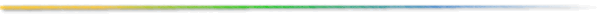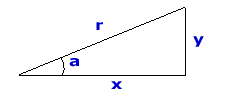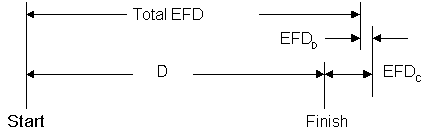## Cycling Science

Power, speed, equivalent flat distance

Three forces act on a rider to oppose forward motion: air resistance, rolling resistance and gravity force. On level ground rolling resistance at predominates at speeds less than 9 mph. At 9 to 10 mph air resistance is equal to rolling resistance. As the rider goes faster, air resistance rises rapidly. At 18 mph it is 4 times greater than rolling resistance, nearly 8 times larger at 25 mph, and 16 times greater at 36 mph.

As the road kicks upward, gravity forces can pull a rider backward with the force of a headwind gale. Air and gravity forces are equal for a typical rider at a speed of 16 mph on a slope of just 1.25%. A headwind of 10 mph has the same effect on your forward speed as a 2% climb. Headwinds of 20 and 30 mph are equivalent to climbing gradients of 4% and 6.4%, respectively.

Air Resistance
Fair = ½ Area CoefDrag Dair Vair²

where:

Area = Cross-sectional area of the rider in m²

Dair = Air Density, kg/m³. Varies with temperature, barometric pressure, and altitude. Can be calculated like this:
Dair = P / (R T)
where:
P = pressure, Pascals (multiply mb by 100 to get Pascals)
R = gas constant, J/(kg * °K) = 287.05 °K for dry air;
T = temperature, °K = °C + 273.15

CoefDrag = Drag coefficient, dimensionless

Vair = Apparent velocity of the wind on the front of a moving rider, m/s. For instance, if the rider is moving with a 5 m/s ground speed (V) into a 2 m/s headwind (Vhw), then Vair = 7 m/s. In other words Vair = V + Vhw.

Rolling Resistance
Froll = 9.8 W CoefRoll

where:

W = Weight of the rider and bike, kg

CoefRoll = Coefficient of rolling resistance, dimensionless (wooden track = 0.001, smooth concrete = 0.002, asphalt road = 0.004, rough paved road = 0.008)

Gravity Force

where:

W = Weight of the rider and bike, kg

grad =Gradient of the climb or descent. More specifically it is the sin(a) which equals y/r. Note: it is not y/x but admittedly this distinction is important only when the angle is greater than about 10° (1.5% error at 10° and 3.5% off at 15°).

Rider Power
Now that we defined the three forces on a rider, we can now use them in an equation that computes rider power.
P = (Fair + Froll + Fgrad) V

where:

V = Ground speed of the rider, m/s

The force of the wind is critical to computing speed or power, but Area and CoefDrag can be hard to estimate. A good technique is to get known power and speed measurements from a bike equiped with a power meter, such as SRM or PowerTap. Then you can solve for the product of (Area * CoefDrag). Then use this product for all of your other calculations.

If you don't have a access to a power meter, perhaps you have a friend who does. Ask him for his average power and speed on a flat course on aerobars and without. You can then calculate his (Area * CoefDrag) product. Estimate yours using the ratio of your weights (bike and rider). That is multiple his by the ratio (Wyou / Wfriend).

Determining velocity from power
The above equations are fine for calculating power given velocity, but what if you want to find the velocity for a given power. In that case you want solve for the velocity V (ground speed of the rider).

To get that we have to expand the power equation like this

P = Q Vair² V + R V

where:
Q = ½ Area CoefDrag Dair
R = 9.8 W (CoefRoll + grad)

and substitute for Vair using the following equation:

Vair = V + Vhw

where:
V = Ground speed of the rider.
Vhw = Headwind. The speed of the wind on the face of a stationary rider.

Simplifying leads to the following equation

0 = Q V³ + 2 Q Vhw V² + (Q Vhw² + R) V - P

This is known as a cubic equation and is notoriously difficult to solve since we have to find its three roots. Sometimes the roots are all real other times only one is real and the other two are imaginary. If there are imaginary roots the single real root is the velocity we're looking for. When all three roots are real our velocity is the maximum one. Fortunately this very excellent website shows how to solve it. You just have to have to arrange your equation in the form:

0 = a V³ + b V² + c V + d

Then plug the values {a, b, c and d} into a series of relatively simple equations to get the velocity of the rider. These equations can easily be solved using your favorite spreadsheet. In our case the values are:

a = Q
b = 2 Q Vhw
c = Q Vhw² + R
d = -P

This calculator does the math for you. Alternatively you can use this spreadsheet.

Equivalent flat distance of a route with climbs and descents
Let's derive an equation that determines the flat miles equivalent to a given climbing height. Then we can just compare the equivalent flat miles (Total EFD) of two routes to determine which is harder. That is, which route takes longer to complete when ridden at the same power.Figure 1: Depiction of the total equivalent flat distance (Total EFD)

What we need is a distance correction for the climbing to add to the route distance and a corresponding one for descending to subtract as shown in Figure 1. This yields the equation:

Eq 1:  Total EFD = D + EFDC + EFDD
where:

Total EFD = Total Equivalent Flat distance. This is the benchmark to compare diverse routes.

D = The measured distance of the route.

EFDC = Climbing Equivalent Flat distance. The extra flat distance that is equal to the route's climbing difficulty. In other words, if you flatten-out all the route's climbing, this is the flat distance that must be added to keep the finishing time the same.

EFDD = Descending Equivalent Flat distance. The flat distance that is equal to the route's descending. In other words, if you flatten-out all the route's descending, this is the flat distance that must be added to keep the finishing time the same. This should alway be negative (adding a negative number is the same as subtracting), since descending should always be faster that riding on the flat.

For bevity several intermediate steps that leading to Eq 3 are skipped. The intermediate steps are shown here. Eq 2 expands to...

Eq 2a: Total EFD = D +
((V0/Vc) - 1) * Dc +
((V0/Vd) - 1) * Dd

where:

V0 = Velocity on the flat (0% gradient) at the same power applied to the climbs.

Vc = Velocity on a climb of a given gradient

Vd = Velocity on a descent of a given gradient

Dc = Climbing distance (DeLorme Topo USA calls it "Climbing Distance"). Total terrestrial distance going uphill.

Dd = Descending distance (DeLorme Topo USA calls it "Descending Distance"). Total terrestrial distance going downhill.

If you have mapping software like Topo USA you have everything you need, otherwise you may have to estimate some of the parameters.

For instance, if the course start and finish are the same location, then the climbing and descending elevations are the same. Then Dc can be estimated as 40% of the route length for very hilly routes, but perhaps 25% or less each for a relatively flat terrain such as here on the east coast of Florida.

Next you need to estimate of the average power that will be applied on the climbs. If you are a course designer this will be the average power of a typical rider expected to rider the route. Keeping in mind the route distance, the average power will be less for a 100-mile route than a 25-miler (perhaps 30 to 40% less). Also your descending power should always be less than your climbing power to account for the inevitable coasting, soft pedaling, etc.

The velocities (V0, Vc and Vd) can be calculated for the power levels choosen and gradients determined using this online Speed Calculator, use a more manual process using this on-line form.

### Alternatively you could write...

Eq 2b:  Total EFD = D + (FCR * Ec ) - (FDR * Ed )

where:

FCR = Flat distance ratio for climbing, dimensionless. This is the flat distance equivalent per unit of climbing. For example if this value 15, then one mile of climbing equals 15 miles of flat distance (or one foot of climbing equals 15 feet of flat distance). See below for how this is computed.

Ec = Climbing elevation. The cummulative height of climbing on the route. DeLorme Topo USA calls this "Climbing Elevation".

FDR = Flat distance ratio for descending, dimensionless. Calculation is similar to FCR.

Ed = Descending elevation. The cummulative height of descending on the route. DeLorme Topo USA calls this "Descending Elevation".

Equivalent flat distance of a climbFigure 2: Breakdown of distances of a route

From figure 2 we can see:

Total EFD = D + EFDC + EFDD

where:

Dd, Df, and Dc are the Descending, Flat, and Climbing distances respectively.

Total EFD = Total Equivalent Flat distance. This is the benchmark to compare diverse routes.

D = The measured distance of the route.

EFDC = Climbing Equivalent Flat distance. The extra flat distance that is equal to the route's climbing difficulty. In other words, if you flatten-out all the route's climbing, this is the flat distance that must be added to keep the finishing time the same.

EFDD = Descending Equivalent Flat distance. The flat distance that is equal to the route's descending. In other words, if you flatten-out all the route's descending, this is the flat distance that must be subtracted to keep the finishing time the same.

The rest of this section I'll derive EFDC. In fact, EFDD turns out to be essentially the same equation.

By definition...

TEFDc = Dc + EFDc

where:

TEFDc = Total Climbing Equivalent Flat distance. Total distance that can be ridden on the flat, in the same time that the rider spends climbing on the route at the same power.

Dc = Distance or length of the route were the rider goes uphill (experiences a gradient > 0). DeLorme Topo USA calls this "Climbing Distance".

EFDC = Extra flat distance due to Climbing. The extra flat distance that is equal to the route's climbing difficulty. In other words, if you flatten-out the climbs, this is the distance that must be added to the climbing distance to get the total flat distance of the climbs.

rearranging...

EFDc = TEFDc - Dc

substituting for TEFDc (by definition it must be Tclimbing * V0)...
EFDc = (Tclimbing * V0) - Dc

where:

Tclimbing = Time that the rider would spend on the climbs

V0 = Velocity on the flat (0% gradient) at the same power applied to the climbs.

substituting for Tclimbing ...
EFDc = ((Dc / Vc ) * V0) - Dc

where:

Vc = Velocity on a climb of a given gradient

rearranging...
Eq A: EFDc = ((V0 / Vc ) - 1) * Dc

This is the equation that we plugged into Eq 1 to derive Eq 2a above.

Flat distance per 1000 ft of climbing
Let's derive an equation that computes the equivalent flat miles for 1000 ft of climbing and flat kilometers per 1000 meters of climbing. Then we can plot them for typical riders.

substituting for Dc...
EFDc = ((V0 / Vc ) - 1) * (Ec / mc)

where:

Ec = Total height of climbs (in the same units as Dc). DeLorme Topo USA calls this "Climbing Elevation".

mc = Average gradient of climbing on the route. It may be estimated or calculated like this: Ec / Dc (again DeLorme Topo USA gives both of these numbers: "Climbing Elevation" and "Climbing Distance", respectively).

rearranging ...
EFDc / Ec = ((V0 / Vc) - 1) / mc

substituting...
Eq B: FCR = ((V0/Vc ) - 1) / mc

where:

FCR = Flat distance ratio for climbing, dimensionless. This is the flat distance equivalent per unit of climbing. For example if this value 15, then one mile of climbing equals 15 miles of flat distance (or one foot of climbing equals 15 feet of flat distance).

V0 = Velocity on the flat (0% gradient) at a given power

Vc = Velocity on the climb (at the same power used to determine V0)

mc = Gradient of the climb (rise/run)

One problem with equation Eq B is that units of climbing and distance are usually different (feet and miles, or meters and kilometers, respectively). To make it more useful we'll modify it slightly for english units.

Eq C: FCRe = FCR (1000/5280)

where:

FCRe= English units, flat miles that are equivalent to 1000 feet of climbing

or if you prefer metric,

Eq D: FCRm = FCR (100/1000)

where:

FCRm = Metric units, flat kilometers that are equivalent to 100 meters of climbing